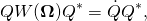### Quaternion evolution

In this note I will repeat some stuff that I was already explaining in the past, but we will also find some important new stuff.
The time evolution differential equation for the attitude matrixis

(1)whereis the angular velocity vector in the body frame, andmaps any vectorinto skew-symmetric matrix as follows:

(2)It has the property that for any vectorwe have

(3)Equation (1) follows immediately from the definition of the angular velocity. The angular velocityin the laboratory frame is defined by

(4)Butmaps coordinates of vectors in the body frame into their coordinates in the laboratory frame. Thusand therefore

(5)But from Eq. (3) we havethereforeand so Eq. (1) follows.
While description of rotations in terms of orthogonalmatrices in principle suffices in classical mechanics, sometimes it is convenient to use the group of quaternions of unit norm, the group isomorphic to the groupused in quantum mechanical description of half-integer spin particles. Quaternions have some advantages in numerical procedures (as for instance in 3D computer games), but they are also convenient for graphical representation of the intrinsic geometry of the rotation group. And this is what interests us, when we plot trajectories representing history of a spinning rigid body.

Quaternions of unit norm, representing rotations, form a 3-dimensional sphere in 4-dimensional Euclidean space, and we are projecting stereographically this sphere onto our familiar three-dimensional space, where we orient ourselves in a usual way known from everyday experience.
The question therefore arises: how the equation describing the time evolution looks like when represented in the quaternion setting?
To derive it we have to return to the fundamental relation between quaternions and rotations of vectors in space.
For every vectorwith componentsdenote bythe pure imaginary quaternion defined as:

(6)Then to unit quaternionthat is such thatthere corresponds rotation matrixsuch that for allthe following identity holds

(7)The second interesting property of the hat map is that for allwe have

(8)whereis the commutator. The property follows directly form the definitions and from the quaternion multiplication rules. Every pure imaginary quaternion is of the formfor someDerivation:
As shown on plaque by the Royal Canal at Broome Bridge in Dublin,the essence of the quaternions is contained in the four equations

(9)From these, multiplying from the left and/or from the right byororwe derive

(10)From the definition of the hat map we have

(11)ThereforeWhen we expand the parenthesis and use the multiplication rules in Eqs. (9),(10), we find that the terms with squares cancel out, while the terms with products likeetc. can be organized as followsThe coefficients in the parentheses on the right are now exactly the components of the cross productThusSuppose now thatis a trajectory in the space of unit quaternions, whileis the corresponding trajectory in the rotation group. Thus we have

(12)for alland allWe now differentiate both sides with respect toOn the left we use the standard product rule for differentiation, but we pay attention so as to preserve the order, because multiplication of quaternions is non commutative. On the right we enter with the differentiation under the “hat”, because it is a linear operation. We obtain:

(13)Assume now thatis a solution of Eq. (1), so thatWe have

(14)We now use Eq. (12) on the right to obtain

(15)In order to get rid ofwe differentiate the defining equation of unit quaternionsto obtain

(16)or

(17)Putting this into Eq. (15) and multiplying both sides on the right bywe obtain

(18)Multiplying from the left by:

(19)From Eq. (16) it follows that the quaternionis pure imaginary:Therefore there exists vectorsuch that

(20)Eq. (19) can now be written as

(21)where on the right we have used Eq. (3). Applying Eq. (8) to the left we end with

(22)Since the above holds for anywe deduce that

(23)Using (20) we finally obtain:

Evolution equation for quaternions

(24)Note: It may be that the final formula can be derived in a shorter way, but I do not know how. It is this last formula that I was using when verifying that the algorithm provided in Meeting with remarkable circles gives indeed a solution of the evolution equation.